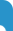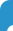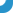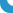## اعضا - نسرین اقبالیفهرست بخش هانسرین اقبالی | برگشت به صفحه کارنامه علمی | [نسخه ویژه چاپ]مقالات چاپ شده E. Ansari-Piri and N. Eghbali, A note on multiplicative and almost multi- plicative linear maps, Honam Mathematical Journal E. Ansari-Piri and N. Eghbali, The nearly additive maps, Bull. Korean Math. Soc N. Eghbali, Stability of cubic functional equation in Non-Archimedean L- fuzzy normed spaces, Albanian J. Math. N. Eghbali, Approximately Jordan maps and their stability, International Journal of Mathematics and Computer Sciences A. Zohri and N. Eghbali, Continuity of fuzzy approximate additive mappings, TWMS Jour. Pure Appl. Math N. Eghbali and F. Heidari, Stability of quadratic and pexiderized quadratic functional equations in non-Archimedean fuzzy n-normed spaces,  Journal of Nonlinear Evolution Equations and Applications N. Eghbali, Non-Archimedean fuzzy versions of approximate ring homomorphisms and derivations, and Derivations, Thai Journal of Mathematics N. Eghbalia and B. Farhadinia, The stability of fuzzy approximately Jordan mappings, Proyecciones Journal of Mathematics E. Ansari-Piri and N. Eghbali, Almost n-multiplicative maps, African Journal of Mathematics and Computer Science Research N. Eghbali and M. Ganji, Hyers-Ulam-Rassias stability of functional equations in Menger probabilistic normed spaces, Journal of Applied Analysis and Computation E. Ansari-Piri and N. Eghbali, The stability of sine and cosine equation, Far East Journal of Mathematical Sciences    E. Ansari-Piri, N. Eghbali and S. Nouri, A note on Jordan mappings between Banach algebras, Far East Journal of Mathematical Sciences    E. Ansari-Piri and N. Eghbali, The stability of Almost n-multiplicative maps in fuzzy normed spaces, World Academy of Science Research and Technology    M. Ganji, N. Eghbali and M. Azimian, Bayes and empirical Bayes estimation of parameter k in negative binomial distribution, Journal of Hyperstructures    N. Eghbali, Ideal convergence of sequences in L-fuzzy non-Archimedean normed spaces, TWMS Journal of Pure and Applied Mathematics   N. Eghbali, Frechet differentiation between Menger probabilistic normed spaces, Proyecciones Journal of Mathematics   N. Eghbali , The stability of approximately mappings in the intuitionistic fuzzy normed spaces,  Palestine Journal of Mathematics   N. Eghbali and M. Ganji,  Generalized statistical convergence in the non-Archimedean L-fuzzy normed spaces, Azerbaijan Journal of Mathematics   M. Ganji, N. Eghbali and F. Gharari, Some fractional special functions and fractional moments, General Mathematics Notes   N. Eghbali and S. Hazrati, On the stability of (alpha,beta,gama)-derivations on Lie C*-algebra associated with a pexiderized quadratic type functional equation,  Communications of the Korean Mathematical Society   N. Eghbali, Stability and super stability of fuzzy approximately *-homomorphisms, Communications Faculty of Sciences, University of Ankara Series A1: Mathematics and Statistics   N. Eghbali, J. M. Rassias and M. Taheri, On the stability of a k-cubic functional equation in intuitionistic fuzzy n-normed spaces, Results in Mathematiccs N. Eghbali, Stability and superstability of fuzzyapproximately ring homomorphisms and fuzzy approximately ring derivations, Journal of Environmental Treatment Techniques N. Eghbali, Hyers- Ulam- Rassias stability of power series equation, Konuralp Journal of Mathematics N. Eghbali and F. Arkian, On uniform fuzzy direct Method for stability  of functional equations, Journal of Mathematical Extension   N. Eghbali and S. Haztrati, Stability of  (alpha,beta,gama)-derivations and homomorphisms on the Lie C*-algebras, Transactions on Algebra and its Applications    N. Eghbali, J. M. Rassias and V. Kalvandi, A fixed point approach to the Mittag-Leffler-Hyers-Ulam stability of a fractional integral equation, Open Mathematics   J. Brzdek and  N. Eghbal, On approximate solutions of some delayed fractional differential equations, Applied Mathematics Letters   N. Eghbali, Stability of pexiderized quadratic functional equation in non-Archimedean L-fuzzy normed spaces, Novisad Journal of Mathematics N. Eghbali, Hyers-Ulam-Rassias type stability of polynomial equations, Konuralp Journal of Mathematics N. Eghbali, Generalized Statistical Convergence in the Non-Archimedean L-fuzzy Normed Spaces, Azerbaijan Journal of Mathematics N. Niamoradi, N. Eghbali and A. A. Noori, On a fractional advection dispersion equation in RN involving a critical nonlinearity, Tbilisi Mathematical Journal N. Eghbali and S. Hazrati, STABILITY OF (α, β, γ)−DERIVATIONS AND HOMOMORPHISMS LIE C∗ -ALGEBRAS, Transactions on Algebra and its Applications N. Eghbali and V. Kalvandi, Mittag-Leffler-Hyers-Ulam stability of Hadamard type fractional integral equations, International Balkan Journal of Mathematics N. Eghbali and V. Kalvandi, A Fixed Point Approach To The Mittag-Leff­ er-Hyers-Ulam Stability Of  Di¤erential Equations y0(x) = F(x; y(x)), Applied Mathematics E-Notes